# Gaseous State

## THE GASEOUS STATE

Characteristics: -
1. Intermolecular space is high.
2. Intermolecular force of attraction is low.
3. Volume is not fixed and shape is not constant.
4. Highly compressible.
5. Evenly mix in all proportions.

## Law of Gaseous Molecules

• Boyle’s law
• Charles law
• Gay lussac’s law

### BOYLE’S LAW:

At constant temperature, pressure of a gas is inversely proportional to volume of that gas.
i. e. $P \alpha \frac {1}{V}$
Or
$\frac {P_1}{P_2}= \frac {V_2}{V_1}$
P1 V1 = P2 V2

#### GRAPHICAL REPRESENTATION

Pressure Volume curve at constant temperature is known as isotherm.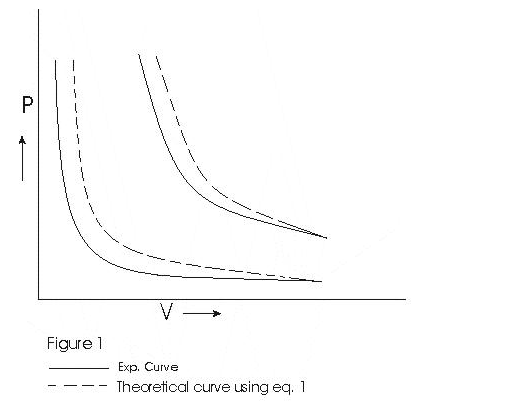Graph above shows that Boyle's law is strictly not obeyed by gases at all values of P and T but it obeys this law only at low pressure and high temperature i.e., at low density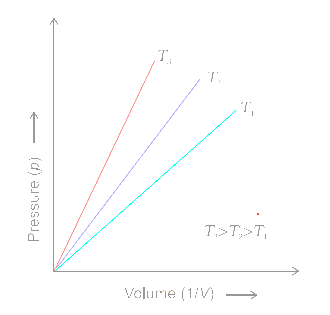The above graph is between P and 1/V
Question
A weather balloon has a volume of 175L when filled with hydrogen gas at pressure of 1 bar. Calculate the volume of balloon when it rises to a height where atmospheric pressure is 0.8 bar. Assume that temp. is constant.
P1 V1 = P2 V2
So, V2 = 175/.8L

### CHARLIE ‘S LAW

At constant pressure, volume of a gas is directly proportional to the temperature.
Mathematically: -
V/T = Constant
Or
V1 /T1 = V2/T2

#### GRAPHICAL REPRESENTATION

Isobar: - The volume temperature curves at constant pressure are known as Isobars.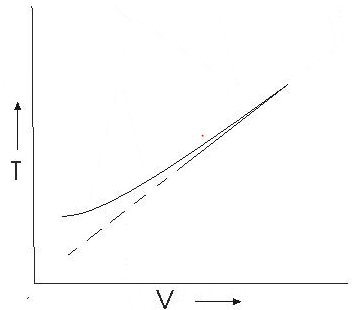This graph shows that experimental graph deviates from straight line. Theoretical and experimental graphs agree at high temperature.

### Absolute Zero

The hypothetical temperature at which gases are supposed to occupy zero volume is known as absolute zero temp.
Question
A certain amount of gas at 27o C and 1 bar pressure occupies volume of 25 m3. If pressure is kept constant & temperature is raised to 77o C, what will be the volume of gas?
$\frac {V_1}{V_2} =\frac {T_1}{T_2}$
$\frac {25}{V_2} =\frac {300}{350}$
V2 =29.16 m3

Question
A flask was heated from 27 to 227o C at constant pressure. Calculate the volume of flask if 0.1 dm3 of air measured at 227o C was expelled from the flask.
Let V be the volume of Flask
$\frac {V_1}{V_2} =\frac {T_1}{T_2}$
$\frac {V}{V+.1}=\frac {300}{500}$
V = 0.15 dm3

### AVOGARD’S Law

At constant temperature and pressure equal volume of all gases contain equal no. of moles or moles.
Mathematically: -
$V \alpha n$
1)The number of molecules in one mole of a gas has been determined to be 6.022 × 1023 and is known as Avogadro constant
2) one mole of each gas at standard temperature and pressure (STP) will have same volume.Standard temperature and pressure means 273.15 K (0°C) temperature and 1 bar (i.e., exactly 105 pascal) pressure. At STP molar volume of an ideal gas or a combination of ideal gases is 22.71098 L mol-1.

### GAY LUSSAC ‘S LAW

At constant volume pressure of a given mass of a gas is directly proportional to the temperature.
Mathematically: -
$P \alpha T$
So, P/T =constant

#### GRAPHICAL REPRESENTATION

Isochors --- The pressure - temperature curve at constant volume are known as Isochors.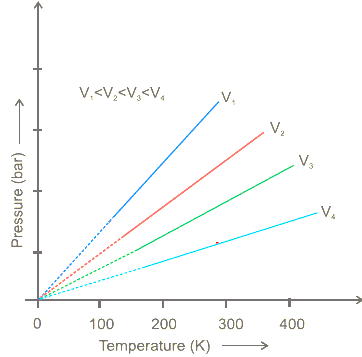## Ideal Gas Equation

### Ideal Gas

A gas that follows Boyle’s law, Charles law & Avogadro’s law strictly is known as an ideal gas.Real gases follow these laws under specific conditions: -
Ideal gases equation
According to Boyle's Law $V \alpha \frac {1}{P}$
According to Charles Law $V \alpha T$
According to Avogadro Law $V \alpha n$
On Combining
$V \alpha \frac{nT}{P}$
$P V \alpha nT$
P V = n R T
R = Universal gas constant
and equation is called Ideal gas equation
R=8.314 J/K moles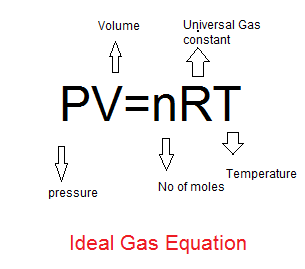If temperature, volume and pressure of a fixed amount of gas vary from T1, V1 and p1 to T2, V2 and p2 then we can write
$P_{1}V_{1}=nRT_{1}$ or $\frac {P_1V_1}{T_1}=nR$
$P_{2}V_{2}=nRT_{2}$ or $\frac {P_2V_2}{T_2}=nR$
or
$\frac {P_1V_1}{T_1}=\frac {P_2V_2}{T_2}$

This is a very useful equation. If out of six, values of five variables are known, the value of unknown variable can be
calculated from the equation This equation is also known as Combined gas law

## Density and Molar Mass of a Gaseous Substance

P V = n R T
n= PV/RT
m/M = PV/RT
(m/V)(1/M) = P/RT
Now d=m/V
d/M=P/RT
or
M=dRT/P
Or
PM= dRT